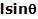Wednesday, March 11, 2009

Irodov Problem 1.283Consider a stationary observer observing the top's motion. The figure depicts the picture of a top spinning while undergoing precession. Under precession the spin angular velocity vector w rotates as shown in the figure. Suppose that angular velocity of precession is w', this means that the angular velocity vector w rotates with an angular velocity w'. In other words the angular momentum vector of the top can be written as,About point O (point of contact of the top with the ground), there is only one torque acting on the top namely due to the force of gravity mg acting at the CG. As shown in the figure the CG is at a distancefrom point O, the torque due to gravity is given by. The net torque must be equal to the rate of change of angular momentum of the top. Thus we have,(b) As seen by an observer located at the CG of the top, there are two forces acting in the horizontal direction i) the centrifugal force as the CG rotates in a circle of radiusat a rate w' given byand ii) the horizontal component of the reaction from point of contact NH. Since from the observers point of view there is no acceleration for the CG, we have,Similarly, it is clear that the vertical component of the reaction from floor at the point of contact must be mg to balance the force of gravity.Anonymous said...
This comment has been removed by a blog administrator.Hi,
I have a doubt in problem of spinning top precession. The centre of mass of spinning top is now rotating in a circle of radius r’=l*sin(theta).
This means there has to be a Centripetal force mr’.w’.w’, this horizontal force is provided by point of contact. But this force will also have a torque on the top which
Should add up with torque due to its own weight… but this term does not appear in solution. May be we can neglect this term if w’ is too small.
But yes, the solution provided by you matches with the one in book :-)
I tried to search this on internet but dint find anything, can u send me ur e-mail id , mine is pradyumn@gmail.com, it will be faster to communicate that way

Thanks